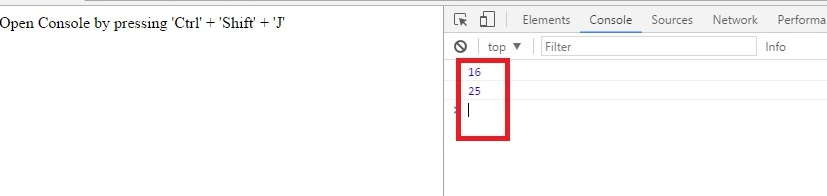# JavaScript program to create a power function and print the result

JavaScript program to create a power function that accepts two parameters, base and exponent and prints the final result.
Submitted by Abhishek Pathak, on June 06, 2017

Power is a very useful functional property in Mathematics. We often require to compute power of a base to an exponent. Why not create a one? It will be simple but with a default option. If a user passes a second parameter, the power will calculate the base power exponent. If the user doesn’t provide second argument, default base to the power 2 will be calculated.

In JavaScript, we are allowed to pass any number of parameters during function call. That said, when we pass less arguments, the subsequent arguments are given with undefined value; when we pass more arguments, the extra arguments are simply ignored.

JavaScript power function:

```function power(base, exponent) {
var result = 1;
if(exponent == undefined)
exponent = 2;
for(var i=1; i<=exponent; i++) {
result = result * base;
}
return result;
}

console.log(power(2,4));
```

Firstly, we define the function. We take a result variable and assign it a value of 1.

Now, we check if the value of exponent is undefined, then set exponent to 2.

Next, we define a for loop that iterates up to exponent times. Inside loop we update result as result into base. Finally, we return the result.

After then we write this function in console.log to print the returned value in the console.JavaScript code with HTML

```<html>
<title>Javascript Power function</title>

<script>
function power(base, exponent) {
var result = 1;
if(exponent == undefined) {
exponent = 2;
}
for(var i=1; i<=exponent; i++) {
result = result * base;
}
return result;
}

console.log(power(2,4));
console.log(power(5));
</script>

<body>

<p>Open Console by pressing 'Ctrl' + 'Shift' + 'J'</p>

</body>
</html>
```

Demo